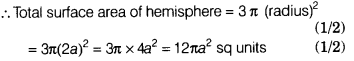# If the radius of a hemisphere is 2 a, then find the total surface area of hemisphere

If the radius of a hemisphere is 2 a, then find the total surface area of hemisphere.

Given, radius of hemisphere = 2a